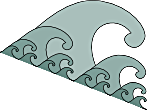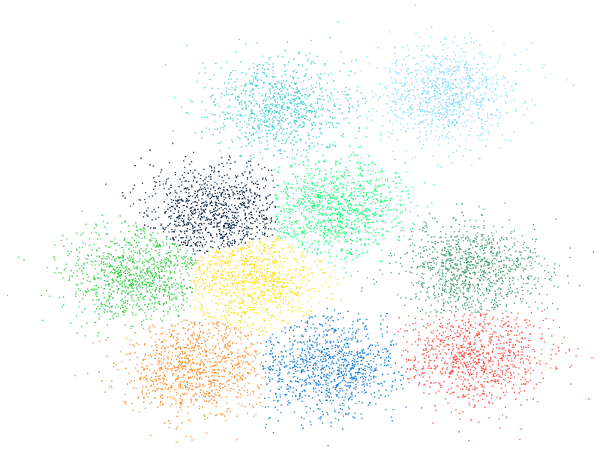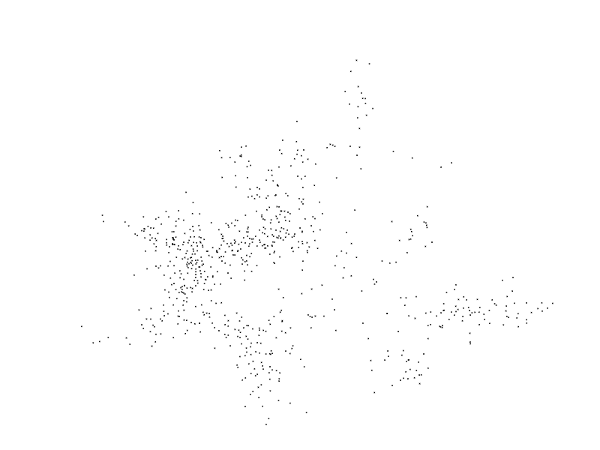# K-averages

K-averages is an iterative hard clustering algorithm that operates in a similar manner to the classic k-means, to which its name is a reference. Compared with the de facto  standard for hard clustering of non-Euclidian data, kernel k-means, k-averages exhibits several distinctive properties:

• It can operate on any symmetrical similarity matrix with guaranteed convergence, no other constraint is imposed.
• It directly finds a set of clusters that constitute a local maximum of the average intra-class similarity, without going through a geometric interpretation of data like the standard kernel k-means does.
• Experimentally, it run 20 times to 100 times faster than kernel k-means, thanks to a much faster convergence rate.
• Tested on a variety of synthetic and natural data, it yields results equivalent to kernel k-means for low numbers of classes (up to 8) and outperforms it for larger numbers.

K-averages has been developed and optimized in Matlab and C; and both versions can be found at https:// bitbucket.org/mlagrange/kaverages.Result of a clustering of 2D Gaussian data produced by kaverages.Repartition of errors relative to ground truth.